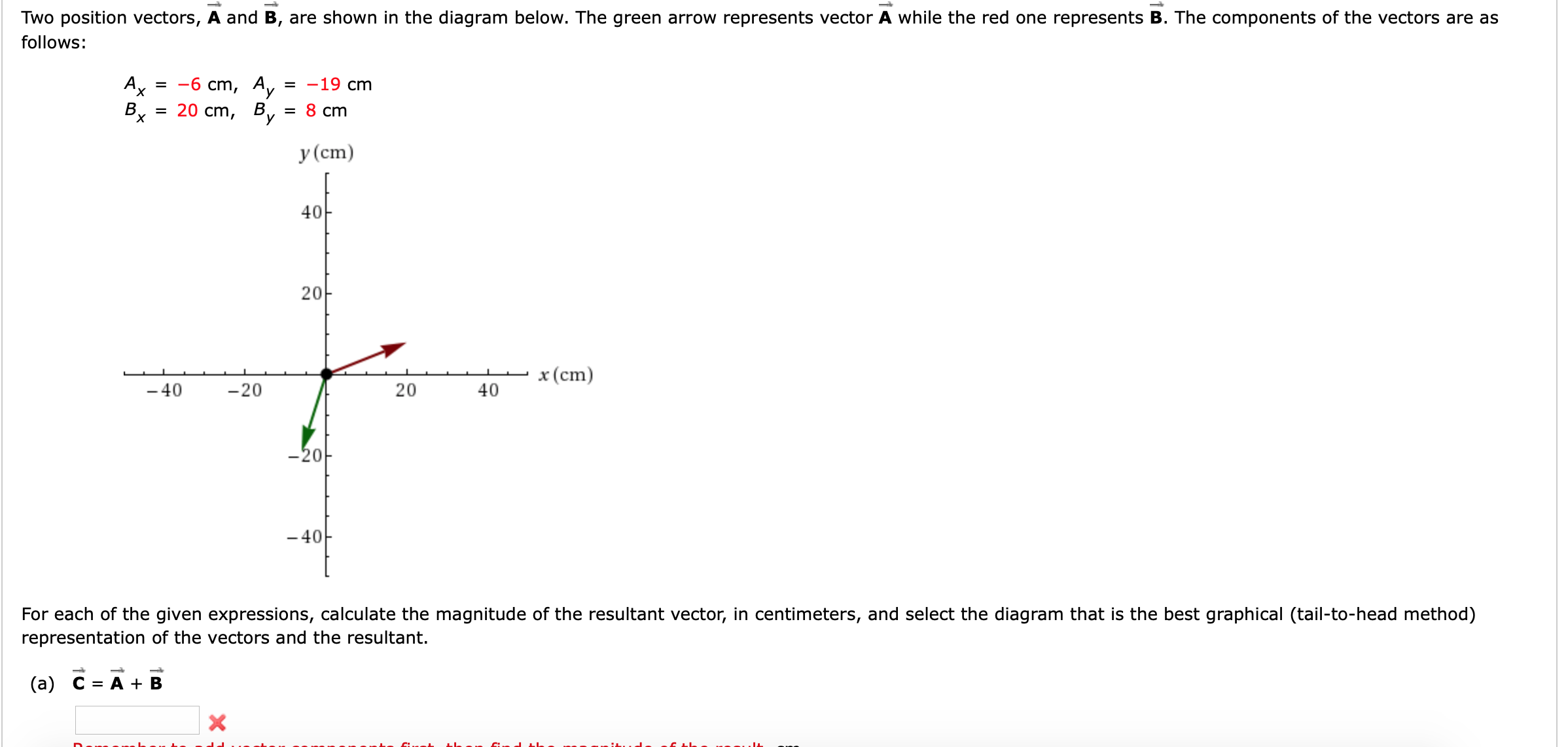# Two position vectors, A and B, are shown in the diagram below. The green arrow represents vector A while the red one represents B. The components of the vectors are asfollows:-6 cm,AxAy-19 cm20 cm, BуВ.х8 cm==у (ст)4020x(cm2040-40-20-20-40For each of the given expressions, calculate the magnitude of the resultant vector, in centimeters, and select the diagram that is the best graphical (tail-to-head method)representation of the vectors and the resultant.(а) С %3D А + В

Question
450 views

Im not sure how to do this question. I included the screen shot. I have attempted and gotten incorrect answers 3 times.help_outlineImage TranscriptioncloseTwo position vectors, A and B, are shown in the diagram below. The green arrow represents vector A while the red one represents B. The components of the vectors are as follows: -6 cm, Ax Ay -19 cm 20 cm, B у В. х 8 cm = = у (ст) 40 20 x(cm 20 40 -40 -20 -20 -40 For each of the given expressions, calculate the magnitude of the resultant vector, in centimeters, and select the diagram that is the best graphical (tail-to-head method) representation of the vectors and the resultant. (а) С %3D А + В fullscreen
check_circle

Step 1

The x component of the vector is,

Step 2

The y component of the vector is,

Step 3

The magnitude of the resultant vector i...

### Want to see the full answer?

See Solution

#### Want to see this answer and more?

Solutions are written by subject experts who are available 24/7. Questions are typically answered within 1 hour.*

See Solution
*Response times may vary by subject and question.
Tagged in

### Vectors and Scalars